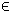## 20L1 Problem

The constrained L1 (L1) problem is defined as

 min x
 Σ i
|ri(x)|
subject to xL x xU
bL Ax bU
cL c(x) cU
(22)
where x,xL,xURn, r(x)RN, c(x),cL,cURm1, bL,bURm2 and ARm2 × n.

The L1 solution can be obtained by the use of any suitable nonlinear TOMLAB solver.

The following files define a problem in TOMLAB.

File: tomlab/quickguide/L1QG_r.m, L1QG_J.m
```  r:   Residual vector
J:   Jacobian matrix
```
The following file illustrates how to solve an L1 problem in TOMLAB. Also view the m-files specified above for more information.

File: tomlab/quickguide/L1QG.m

Open the file for viewing, and execute L1QG in Matlab.
```% L1QG is a small example problem for defining and solving
% an L1 problem using the TOMLAB format.

x_0  = [1;1];        % Initial value
x_L  = [-10;-10];    % Lower bounds on x
x_U  = [10;10];      % Upper bounds on x

% Solve the problem min max |r_i(x)|, where i = 1,2,3
% Solve the problem by eliminating abs, doubling the residuals, reverse sign
% i.e. min max [r_1; r_2; r_3; -r_1; -r_2; -r_3];
y = [-1.5; -2.25; -2.625]; % The data values
t = [];                 % No time vector used

% Generate the problem structure using the Tomlab format
% as a standard least squares problem.
%
% The part in the residuals dependent on x are defined in L1ex_r.m
% The Jacobian is defined in L1ex_J.m

Prob = clsAssign('L1QG_r', 'L1QG_J', [], x_L, x_U, Name, x_0, y, t);

% Set JacPattern, L1Solve will compute correct ConsPattern
Prob.JacPattern  = ones(length(y),2);

% Set the optimal values into the structure (for nice result presenting)

Prob.x_opt=[3, 0.5];
Prob.f_opt=0;

% Use the standard method in L1Solve
Prob.L1Type = 1;

% Get the default solver
Solver = GetSolver('con',1,0);

Prob.SolverL1 = Solver;

% One may set the solver directly:
%Prob.SolverL1 = 'snopt';
%Prob.SolverL1 = 'npsol';
%Prob.SolverL1 = 'minos';
%Prob.SolverL1 = 'conSolve';

% These statements generate ASCII log files when running SNOPT
if strcmpi(Solver,'snopt')
Prob.SOL.PrintFile = 'snopt.txt';
Prob.SOL.SummFile  = 'snopts.txt';
Prob.SOL.optPar(1) = 10;   % Print level in SNOPT
%Prob.SOL.optPar(13) = 3;   % Verify level in SNOPT
end

% Set print level 2 to get output from PrintResult at the end
PriLev = 2;
Prob.PriLevOpt = 0;

Result  = tomRun('L1Solve', Prob, PriLev);```